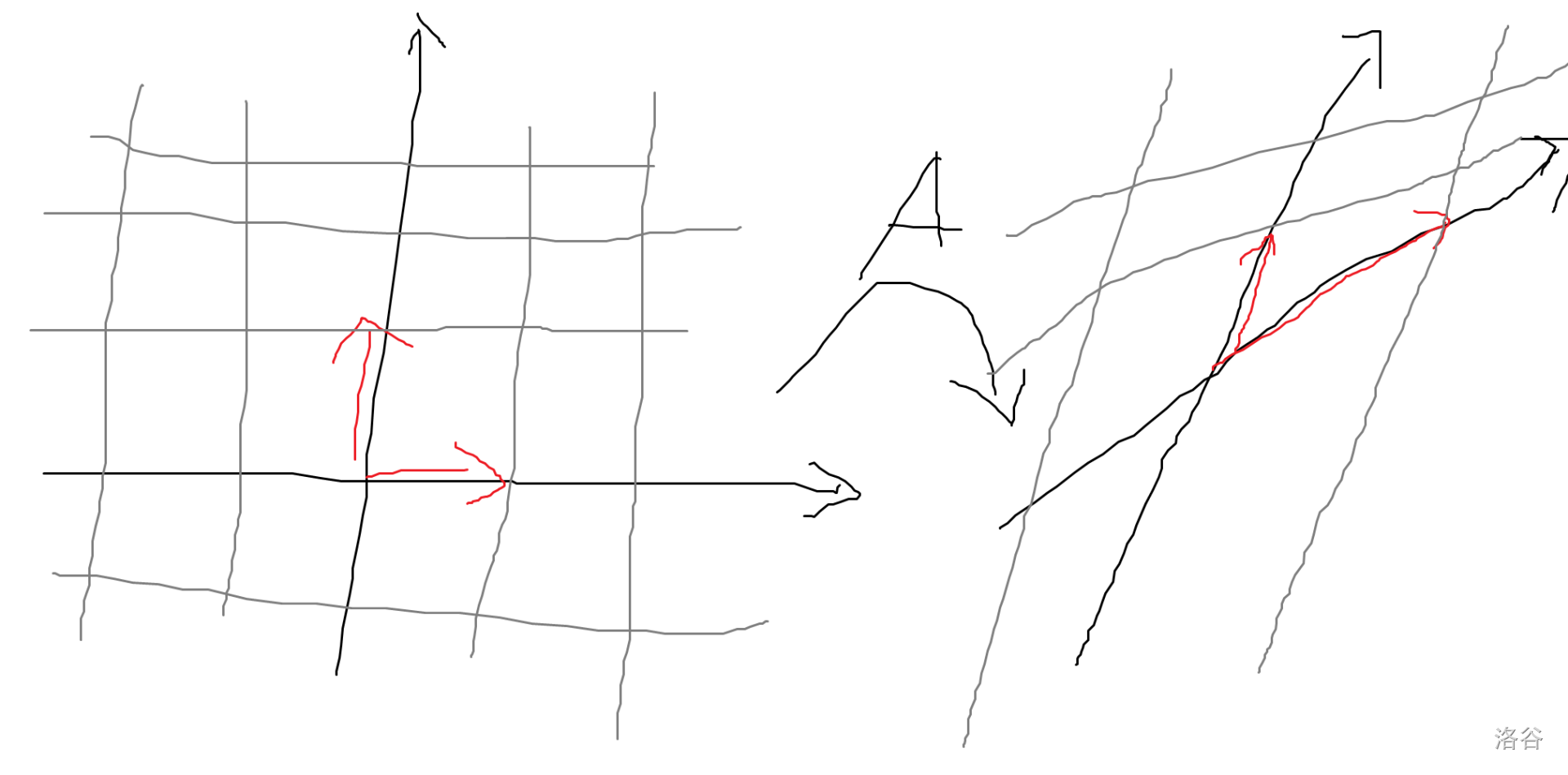# x义x的博客

……就算是单程票，也是可以的吧？

0%

## 基础知识

### 线性相关

• 如果 $X$ 线性相关，那么至少有一个 $X_i$ 是其他向量的线性组合。

（此处并非全部：反例如 $(1,0),(2,0),(0,1)$）

• 如果存在 $X_i$ 是其他向量的线性组合，那么 $X$ 线性相关。

• 如果 $X$ 的一部分线性相关那么 $X$ 也线性相关。

• 如果 $X$ 线性无关那么 $X$ 的任何一部分也线性无关。

• 如果 $X_1,X_2,…,X_n$ 线性无关而 $X_1,X_2,…,X_n,X_{n+1}$ 线性相关，那么 $X_{n+1}$ 是 $X_1,X_2,…,X_n$ 的线性组合。

• 如果 $X_1,X_2,…,X_n$ 线性无关而 $X_{n+1}$ 不能表示为它们的线性组合，那么 $X_1,X_2,…,X_n,X_{n+1}$ 也线性无关。

### 基，维数

（在指出一个向量的坐标前必须先指出基。坐标为 $(1,0,…,0),(0,1,…,0),…,(0,0,…,1)$ 的向量并非坐标恰好很特殊，而是它们定义了坐标。）

（下面我们可能会混淆一个向量和它的坐标，但请记住坐标总是也和基有关。否则学习基变换的时候可能会遇到一些困难。）

## 线性变换

### 线性映射的定义（此处灵魂画手了，原来的网格映射后还是平行的。）

• $(XY)^T=Y^TX^T$
• $\text{rank} (XY)\le \min(\text{rank}\ X,\text{rank}\ Y)$

### 线性变换环

$n$ 相等的所有线性变换构成一个环。

• 如果 $A$ 不退化那么它的转置也不退化。

• 如果 $B$ 不退化，那么 $\text{rank}(AB)=\text{rank}(BA)=\text{rank}\ A$。

• 如果 $A_i$ 均不退化，那么 $(A_1A_2…A_n)^{-1}=A_n^{-1}A_{n-1}^{-1}…A_1^{-1}$。

## 行列式

• $\det A=\det (A^T)$

• 交换 $A$ 的任意两行，行列式变号。（斜对称性

• 可以把行列式运算看成一个对 $A$ 的 $n$ 个行向量/列向量的函数。对于任意一个行向量/列向量它都是线性的。（多重对称性）具体来说：

• 如果某一行/列 $A_i$ 全为 0，那么 $\det A=0$。如果某两行/列相同，那么 $\det A=0$。（从现实意义上可以很容易看出，一个被“拍扁”的立方体体积显然是 0）

• 上两条的推论：如果使 $A_u=A_u+\lambda A_v$ 而生成一个新的矩阵 $A’$（也被称为在 $A$ 上进行行/列初等变换），有 $\det A’=\det A$。（$\det A’$ 可以拆成 $\det A+\lambda \det B$，其中 $B_u=B_v$ 所以 $\det B=0$。）

• 如果将 $A$ 是一个上三角矩阵，那么它的行列式就是对角线元素之积。（这提供了我们一个 $O(n^3)$ 计算行列式的方法。）

• $\det AB=\det A\det B$。

## 行列式的应用

• 一个矩阵不退化等价于其行列式不为 0。

• Cramer 法则）线性方程组